# NCERT Solutions class-11 Chemistry Equilibrium Part-3## myCBSEguide App

Complete Guide for CBSE Students

NCERT Solutions, NCERT Exemplars, Revison Notes, Free Videos, CBSE Papers, MCQ Tests & more.

51. The pH of 0.005M codeine (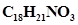) solution is 9.95. Calculate its ionization constant and pKb.

52. What is the pH of 0.001 M aniline solution? The ionization constant of aniline can be taken from Table 7.7. Calculate the degree of ionization of aniline in the solution. Also calculate the ionization constant of the conjugate acid of aniline.

53. Calculate the degree of ionization of 0.05M acetic acid if its pKa value is 4.74.

How is the degree of dissociation affected when its solution also contains (a) 0.01 M (b) 0.1 M in HCl?

54. The ionization constant of dimethylamine is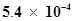. Calculate its degree of ionization in its 0.02 M solution. What percentage of dimethylamine is ionized if the solution is also 0.1 M in NaOH?

55. Calculate the hydrogen ion concentration in the following biological fluids whose pH are given below:

(a) Human muscle-fluid, 6.83

(b) Human stomach fluid, 1.2

(c) Human blood, 7.38

(d) Human saliva, 6.4.

56. The pH of milk, black coffee, tomato juice, lemon juice and egg white are 6.8, 5.0, 4.2, 2.2 and 7.8 respectively. Calculate corresponding hydrogen ion concentration in each.

57. If 0.561 g of KOH is dissolved in water to give 200 mL of solution at 298 K. Calculate the concentrations of potassium, hydrogen and hydroxyl ions. What is its pH?

58. The solubility of Sr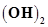at 298 K is 19.23 g/L of solution. Calculate the concentrations of strontium and hydroxyl ions and the pH of the solution.

59. The ionization constant of propanoic acid is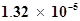. Calculate the degree of ionization of the acid in its 0.05 M solution and also its pH. What will be its degree of ionization if the solution is 0.01M in HCl also?

60. The pH of 0.1M solution of cyanic acid (HCNO) is 2.34. Calculate the ionization constant of the acid and its degree of ionization in the solution.

61. The ionization constant of nitrous acid is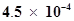. Calculate the pH of 0.04 M sodium nitrite solution and also its degree of hydrolysis.

62. A 0.02 M solution of pyridinium hydrochloride has pH = 3.44. Calculate the ionization constant of pyridine

63. Predict if the solutions of the following salts are neutral, acidic or basic:

NaCl, KBr, NaCN,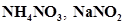and KF

64. The ionization constant of chloroacetic acid is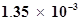. What will be the pH of 0.1M acid and its 0.1M sodium salt solution?

65. Ionic product of water at 310 K is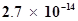. What is the pH of neutral water at this temperature?

66. Calculate the pH of the resultant mixtures:

a) 10 mL of 0.2M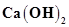+ 25 mL of 0.1M HCl

b) 10 mL of 0.01M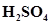+ 10 mL of 0.01Mc) 10 mL of 0.1M+ 10 mL of 0.1M KOH

67. Determine the solubilities of silver chromate, barium chromate, ferric hydroxide, lead chloride and mercurous iodide at 298K from their solubility product constants given in Table 7.9 (page 221). Determine also the molarities of individual ions.

68. The solubility product constant of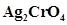and AgBr are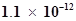and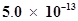respectively. Calculate the ratio of the molarities of their saturated solutions.

69. Equal volumes of 0.002 M solutions of sodium iodate and cupric chlorate are mixed together. Will it lead to precipitation of copper iodate? (For cupric iodate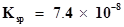).

70. The ionization constant of benzoic acid is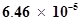and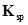for silver benzoate is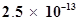. How many times is silver benzoate more soluble in a buffer of pH 3.19 compared to its solubility in pure water?

71. What is the maximum concentration of equimolar solutions of ferrous sulphate and sodium sulphide so that when mixed in equal volumes, there is no precipitation of iron sulphide? (For iron sulphide,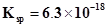).

72. What is the minimum volume of water required to dissolve 1g of calcium sulphate at 298 K? (For calcium sulphate,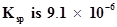).

73. The concentration of sulphide ion in 0.1M HCl solution saturated with hydrogen sulphide is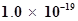M. If 10 mL of this is added to 5 mL of 0.04 M solution of the following: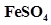,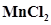,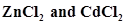. in which of these solutions precipitation will take place?

Given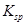for FeS=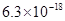. MnS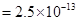,ZnS=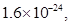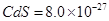## Test Generator

Create Papers with your Name & Logo